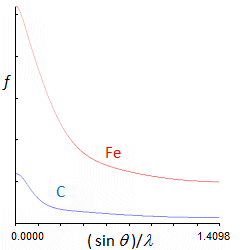# Atomic scattering factor

### From Online Dictionary of Crystallography

Facteur de diffusion atomique (Fr). Formfaktor (Ge). Factor de forma atómica (Sp). Fattore di diffusione atomico (It). 原子散乱因子 (Ja).

## Definition

A measure of the scattering power of an isolated atom. Also known as the atomic form factor. The scattering factor depends on the scattering amplitude of an individual atom, on the Bragg angle and the type of radiation involved.

## X-ray scattering

The scattering from a crystal of an X-ray beam results from the interaction between the electric component of the incident electromagnetic radiation and the electrons in the crystal. Tightly bound electrons scatter coherently (Rayleigh scattering); free electrons scatter incoherently (Compton scattering). The scattering process from atomic electrons in a crystal lattice has both coherent and incoherent components, and is described as Thomson scattering.Scattering factor of stationary C and Fe atoms plotted as a function of Bragg angle for incident X-ray wavelength of 0.70930 Å. Ticks on the horizontal axis correspond to Bragg angle increments of 10 degrees; ticks on the vertical axis are increments of 5 electrons.

The scattering amplitude from a neutral atom depends on the number of electrons (Z = the atomic number) and also on the Bragg angle θ – destructive interference among waves scattered from the individual electrons reduces the intensity at other than zero scattering angle. For θ = 0 the scattering amplitude is normally equal to Z. However, the scattering factor is modified by anomalous scattering if the incident wavelength is near an absorption edge of the scattering element.

The X-ray scattering factor is evaluated as the Fourier transform of the electron density distribution of an atom or ion, which is calculated from theoretical wavefunctions for free atoms.